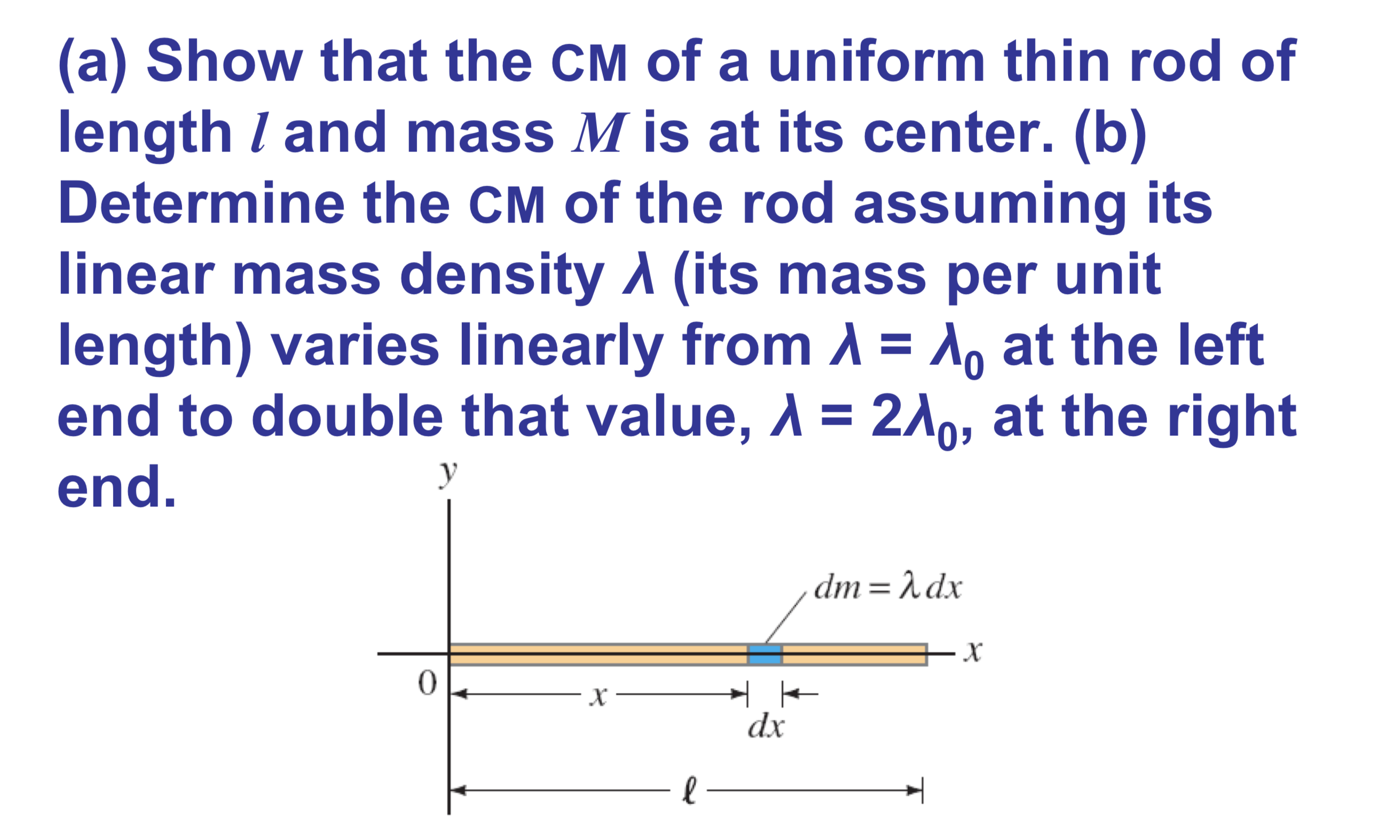# (a) Show that the CM of a uniform thin rod oflength / and mass M is at its center. (b)Determine the CM of the rod assuming itslinear mass density A (its mass per unitlength) varies linearly from A = at the leftend to double that value, A = 2A0, at the rightyend.dm λdxX0хdx

Question
56 viewshelp_outlineImage Transcriptionclose(a) Show that the CM of a uniform thin rod of length / and mass M is at its center. (b) Determine the CM of the rod assuming its linear mass density A (its mass per unit length) varies linearly from A = at the left end to double that value, A = 2A0, at the right y end. dm λdx X 0 х dx fullscreen
check_circle

Step 1

(a)

Consider lambda be the uniform mass density of the rod.

From the definition of center of mass.

Step 2

Total length of the rod is l and the result obtained for the center of mass is l/2. Therefore, required result is proved.

Step 3

(b)

As the linear mass density of the rod is varying. Theref...

### Want to see the full answer?

See Solution

#### Want to see this answer and more?

Solutions are written by subject experts who are available 24/7. Questions are typically answered within 1 hour.*

See Solution
*Response times may vary by subject and question.
Tagged in

### Centre of Mass and Centre of Gravity